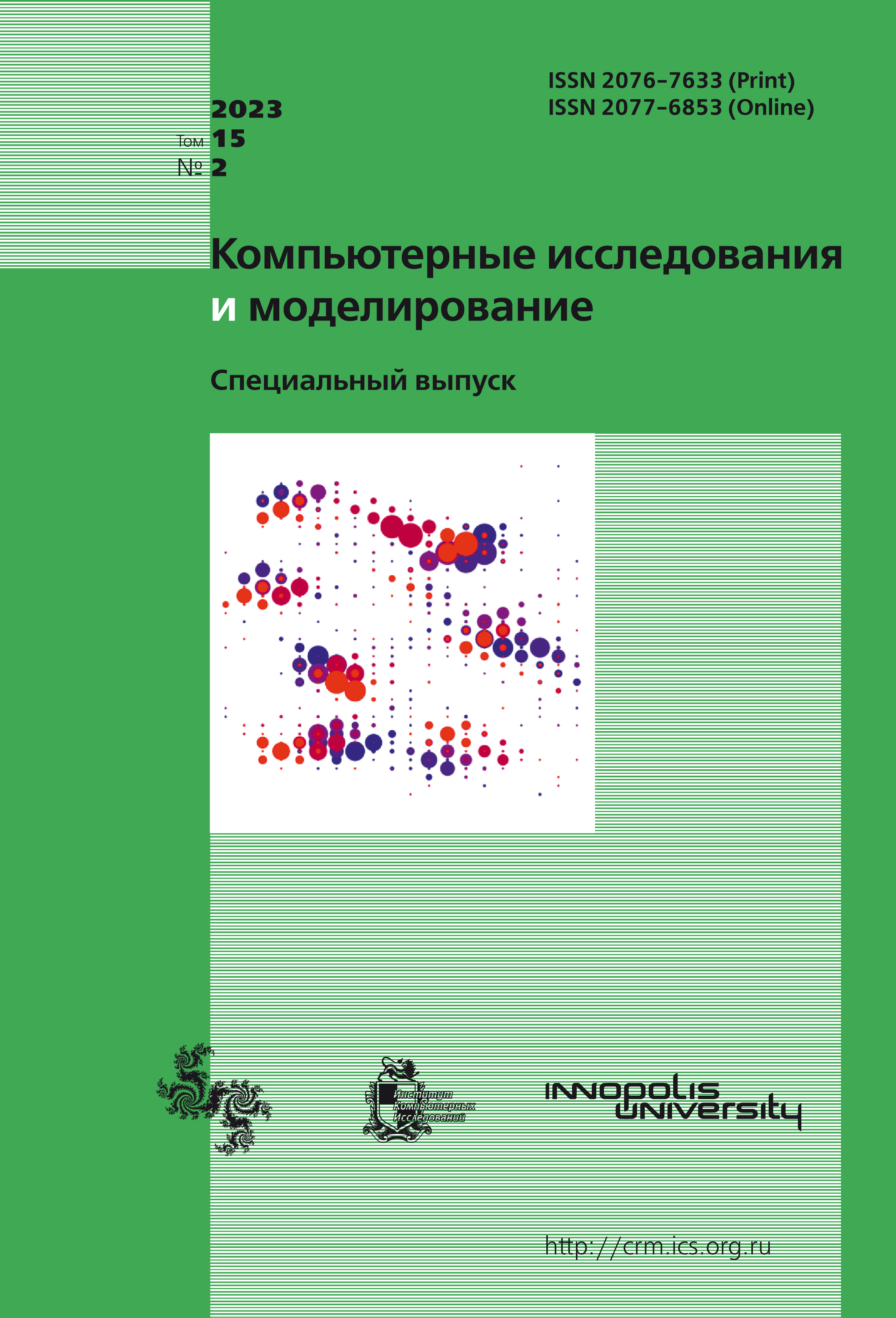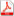Latest issue Issue 2, 2023 Vol. 15

# All issues

Numerical solving of an inverse problem of a hyperbolic heat equation with small parameterpdf (540K)

In this paper we describe an algorithm of numerical solving of an inverse problem on a hyperbolic heat equation with additional second time derivative with a small parameter. The problem in this case is finding an initial distribution with given final distribution. This algorithm allows finding a solution to the problem for any admissible given precision. Algorithm allows evading difficulties analogous to the case of heat equation with inverted time. Furthermore, it allows finding an optimal grid size by learning on a relatively big grid size and small amount of iterations of a gradient method and later extrapolates to the required grid size using Richardson’s method. This algorithm allows finding an adequate estimate of Lipschitz constant for the gradient of the target functional. Finally, this algorithm may easily be applied to the problems with similar structure, for example in solving equations for plasma, social processes and various biological problems. The theoretical novelty of the paper consists in the developing of an optimal procedure of finding of the required grid size using Richardson extrapolations for optimization problems with inexact gradient in ill-posed problems.

Keywords: inverse and ill-posed problems, hyperbolic heat equation, inexact gradient, Richardson method, regularization
Citation in English: Akindinov G.D., Matyukhin V.V., Krivorotko O.I. Numerical solving of an inverse problem of a hyperbolic heat equation with small parameter // Computer Research and Modeling, 2023, vol. 15, no. 2, pp. 245-258
Citation in English: Akindinov G.D., Matyukhin V.V., Krivorotko O.I. Numerical solving of an inverse problem of a hyperbolic heat equation with small parameter // Computer Research and Modeling, 2023, vol. 15, no. 2, pp. 245-258
DOI: 10.20537/2076-7633-2023-15-2-245-258

Full-text version of the journal is also available on the web site of the scientific electronic library eLIBRARY.RU

The journal is included in the Russian Science Citation Index

The journal is included in the List of Russian peer-reviewed journals publishing the main research results of PhD and doctoral dissertations.

International Interdisciplinary Conference "Mathematics. Computing. Education"

The journal is included in the RSCI

Indexed in Scopus